# Vectorization in Python

• Vectorization is a technique of executing operations on entire arrays without using a loop.
• Vectorization helps to speed up the Python code.
• There are various operations are being performed over vector instead of arrays such as Dot Product, Outer Product, Element wise Product.
• The dot product of vectors which is also known as the scalar product as it produces a single output
• The dot product is an algebraic operation in which two equal length vectors are being multiplied and it produces a single number.
• Let’s consider two matrix a and b of the same length, the dot product is done by taking the transpose of the first matrix and then mathematical matrix multiplication of a’(transpose of a) and b is followed as shown in the figure below.
• The tensor product of two coordinate vectors is termed as Outer Product.
• The outer products which result in a square matrix of dimension equal to (length X length) of the vectors.
• Let’s consider two vectors a and b with dimension n x 1 and m x 1 then the outer product of the vector results in a rectangular matrix of n x m.
• If two vectors have the same dimension then the resultant matrix will be square as shown in the figure.
• Element wise multiplication of two matrices is the algebraic operation in which each element of the first matrix is multiplied by its corresponding element in the later matrix.
• The dimension of the matrices should be the same.
• In the below example, consider two matrices a and b, index of an element in a is i and j then a(i, j) is multiplied with b(i, j) respectively as shown in the figure below.

--

--

--

## More from Vaishali Goilkar

Love podcasts or audiobooks? Learn on the go with our new app.

## Defining Infrastructure Declaratively with Crossplane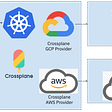## Ethanim Validation Mining and related campaigns will start soon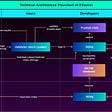## Unwrapping the Permission model in WSO2 API Manager 3.x and 4.x## Refactoring web applications## Golang (GO) pprof in Beego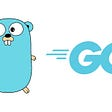## Linux Shell Script 1 Pipe, Redirection & File Descriptor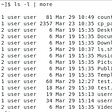## Best Front-end & Back-end Languages to Learn in 2019## Exploring Kafka listeners## Conditional Statements in Python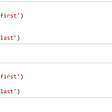## Python Data Structure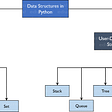## Data Cleaning in Python.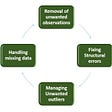## Mastering python for beginner - 1 Installation python